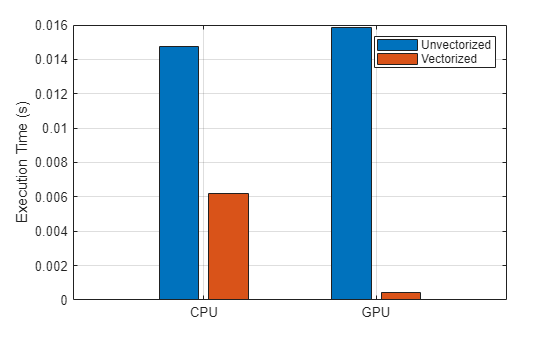# Improve Performance Using a GPU and Vectorized Calculations

This example shows how to speed up your code by running a function on the GPU instead of the CPU and by vectorizing the calculations.

MATLAB® is optimized for operations involving matrices and vectors. The process of revising loop-based, scalar-oriented code to use MATLAB matrix and vector operations is called vectorization. Vectorized code often runs much faster than the corresponding loop-based code and is generally shorter and easier to understand. For an introduction to vectorization, see Using Vectorization.

This example compares the execution time for a function executing on the CPU and the GPU before and after vectorizing the function.

### Time Loop-Based Function Execution on the GPU and the CPU

Fast convolution is a common operation in signal processing applications. A fast convolution operation comprises these steps.

1. Transform each column of data from the time domain to the frequency domain.

2. Multiply the frequency-domain data by the transform of a filter vector.

3. Transform the filtered data back to the time domain and store the result in a matrix.

This section performs a fast convolution on a matrix using the `fastConvolution` supporting function. The function is defined at the end of this example.

Create random, complex input data and a random filter vector.

```data = complex(randn(4096,100),randn(4096,100)); filter = randn(16,1);```

Perform fast convolution on the data using the `fastConvolution` function on the CPU and measure the execution time using the `timeit` function.

`CPUtime = timeit(@()fastConvolution(data,filter))`
```CPUtime = 0.0132 ```

Execute the function on the GPU by changing the input data to be a `gpuArray` object rather than normal MATLAB arrays. As the `fastConvolution` function uses the `'like'` syntax of the `zeros` function, the output is a `gpuArray` if the `data` is a `gpuArray`. To time function execution on the GPU, use `gputimeit`. For functions that use the GPU, `gputimeit` is better that `timeit` for because it ensures that all operations on the GPU finish before recording the elapsed time. The function takes longer to execute on the GPU than on the CPU for this particular problem. The reason is that the `for`-loop executes a fast Fourier transform (FFT), multiplication, and an inverse FFT (IFFT) operation on individual columns of length 4096. Performing these operations on each column individually does not effectively utilize GPU computing power, as GPUs are generally more effective when performing larger numbers of operations.

```gData = gpuArray(data); gFilter = gpuArray(filter); GPUtime = gputimeit(@()fastConvolution(gData,gFilter))```
```GPUtime = 0.0160 ```

### Time Vectorized Function Execution on the CPU and the GPU

Vectorizing code is a straightforward way to improve its performance. You can vectorize the FFT and IFFT operations simply by passing all of the data as inputs, rather than passing each column individually within a `for`-loop. The multiplication operator `.*` multiplies the filter by every column in a matrix at once. The vectorized supporting function `fastConvolutionVectorized` is provided at the end of this example. To see how the function has been vectorized, compare the supporting functions `fastConvolution` and `fastConvolutionVectorized`.

Perform the same calculations using the vectorized function and compare the timing results to the execution of the unvectorized function.

`CPUtimeVectorized = timeit(@()fastConvolutionVectorized(data,filter))`
```CPUtimeVectorized = 0.0046 ```
`GPUtimeVectorized = gputimeit(@()fastConvolutionVectorized(gData,gFilter))`
```GPUtimeVectorized = 4.5261e-04 ```
`CPUspeedup = CPUtime/CPUtimeVectorized`
```CPUspeedup = 2.8720 ```
`GPUspeedup = GPUtime/GPUtimeVectorized`
```GPUspeedup = 35.3766 ```
```bar(categorical(["CPU" "GPU"]), ... [CPUtime CPUtimeVectorized; GPUtime GPUtimeVectorized], ... "grouped") ylabel("Execution Time (s)") legend("Unvectorized","Vectorized")```Vectorizing the code improves performance on the CPU and GPU. However, vectorization improves performance on the GPU much more than on the CPU. The vectorized function runs roughly 2.9x faster than the loop-based function on the CPU and roughly 35.4x faster than the loop-based function on the GPU. The loop-based function runs 21.3% slower on the GPU than on the CPU, but the vectorized function runs about 10.1x faster on the GPU than on the CPU.

When you apply the techniques described in this example to your own code, the performance improvement will strongly depend on your hardware and on the code you run.

### Supporting Functions

Perform a fast convolution operation by transforming each column of data from the time domain to the frequency domain, multiplying it by the transform of a filter vector, transforming back to the time domain, and storing the result in an output matrix.

```function y = fastConvolution(data,filter) % Zero-pad filter to the column length of data, and transform [rows,cols] = size(data); filter_f = fft(filter,rows); % Create an array of zeros of the same size and class as data y = zeros(rows,cols,'like',data); for idx = 1:cols % Transform each column of data data_f = fft(data(:,idx)); % Multiply each column by filter and compute inverse transform y(:,idx) = ifft(filter_f.*data_f); end end```

Perform a fast convolution operation, replacing the `for`-loop with vector operations.

```function y = fastConvolutionVectorized(data,filter) % Zero-pad filter to the length of data, and transform [rows,~] = size(data); filter_f = fft(filter,rows); % Transform each column of the input data_f = fft(data); % Multiply each column by filter and compute inverse transform y = ifft(filter_f.*data_f); end```